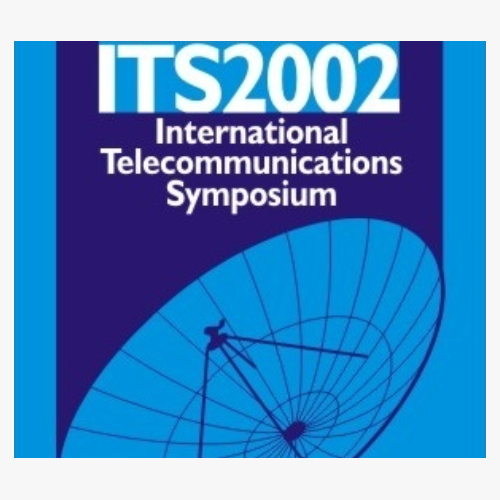#### 2002 International Telecommunications Symposium

Página de 19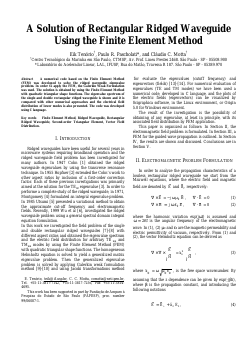##### A Solution of Rectangular Ridged Waveguide Using the Finite Element Method

###### Abstract
"A numerical code based on the Finite Element Method (FEM) was developed to solve the ridged waveguide eigenvalue problem. In order to apply the FEM, the Galerkin Weak Formulation was used. The solution is obtained by using the Finite Element Method with quadratic triangular shape functions. The eigenvalue spectrum of the single and double rectangular ridged waveguide is shown and it is compared with other numerical approaches and the electrical field distribution of lower modes is also presented. The code was developed using C language. "##### Cluster finding algorithm using linear array antenna

###### Abstract
"Fast advance of Array Antenna research ensures its role as a key technology for future cellular systems [1,2]. However, most Smart Antenna techniques are restricted by the assumption that the number of signals is smaller than the array, although cost limits them to small sizes and researches indicate that usual path-distributions do not satisfy such assumption . Alternatively, a good model is that a user\u2019s multipaths arrive at the base Gaussiamly and, according to the Central Limit Theorem, so is the distribution of their crowd. Clearly, a small DOA deviation results when the base station is near a publicly relevant facility where users tend to concentrate while a large one results when users are spread. In order to joint the benefits of code (CDMA) and spatial (SDMA) orthogonality among users, an algorithm that estimate the path-distribution using a small array is urged and is the contribution of this paper."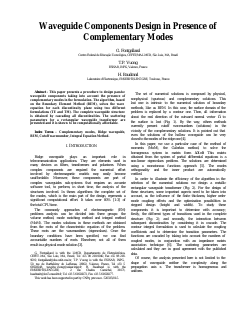###### Abstract
"This paper presents a procedure to design passive waveguide components taking into account the presence of complementary modes in the formulation. The algorithm, based on the Boundary Element Method (BEM), solves the wave equation for each discontinuity plane using two different formulations (TE and TM). The complete waveguide structure is obtained by cascading all discontinuities. The scattering parameters for a rectangular waveguide transformer are presented and it is shown to be computationally affordable."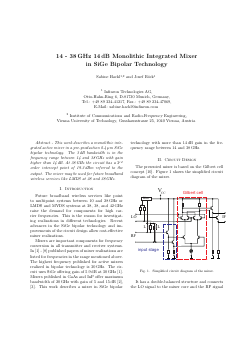##### 14-38 GHz 14 dB Monolithic Integrated Mixer in SiGe Bipolar Technology

###### Abstract
"This work describes a monolithic integrated active mixer in a pre-production 0.4 \u00b5m SiGe bipolar technology. The 3 dB bandwidth is in the frequency range between 14 and 38 GHz with gain higher than 14 dB. At 38 GHz the circuit has a 3rd order intercept point of 19.3 dBm referred to the output. The mixer may be used for future broadband wireless services like LMDS at 28 and 38 GHz."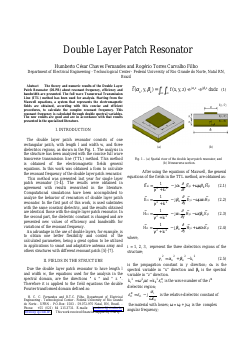##### Double Layer Patch Resonator

###### Abstract
"The theory and numeric results of the Double Layer Patch Resonator (DLPR) about resonant frequency, efficiency and bandwidth are presented. The full wave Transversal Transmission Line (TTL) method has been used for analysis. Starting from the Maxwell equations, a system that represents the electromagnetic fields are obtained, according with this concise and efficient procedures, to calculate the complex resonant frequency. This resonant frequency is calculated through double spectral variables. The new results are good and are in accordance with that results presented in the specialized literature."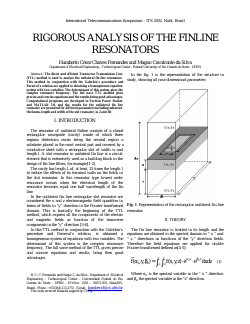##### Rigorous Analysis of the Finline Resonators

###### Abstract
"The direct and efficient Transverse Transmission Line (TTL) method is used to analyse the unilateral fin-line resonators. This method in conjunction with the Galerkin\u2019s procedure and Parseval\u2019s relation are applied to obtaining a homogeneous equation system with two variables. The determinant of this system gives the complex resonance frequency. The full wave TTL method gives precise and concise equations and the results bring good advantages. Computational programs are developed in Fortran Power Station and MATLAB 5.0, and the results for the unilateral fin line resonator are presented for different parameters including substrate thickness, length and width of the slot resonator, in 2 and 3D."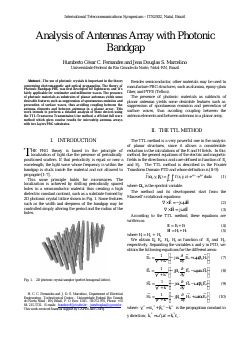##### Analysis of Antennas Array with Photonic Bandgap

###### Abstract
"The use of photonic crystals is important in the theory concerning electromagnetic and optical propagation. The theory of Photonic Bandgap-PBG was first developed for lightwaves and it\u2019s fairly applicable for centimeter and millimeter waves. The presence of photonic materials as substrates of planar antennas yields some desirable features such as suppression of spontaneous emission and prevention of surface waves, thus avoiding coupling between the antenna elements and between antennas in a planar array. This work intends to perform a detailed analysis of these devices using the TTL-Transverse Transmission Line method, a efficient full wave method which gives concise results for microstrip antenna arrays with two layers PBG substrates."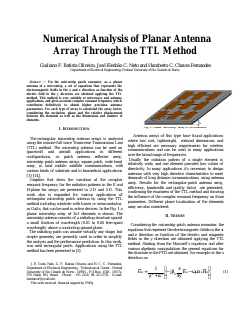##### Numerical Analysis of Planar Antenna Array Through the TTL Method

###### Abstract
"For the microstrip patch resonator, as a planar antenna of a microstrip, a set of equations that represents the electromagnetic fields in the x and z direction as function of the electric field in the y direction are obtained applying the TTL method. This method is very suitable at microwave and antenna applications, and gives accurate complex resonant frequency which contributes definitively to obtain higher precision antenna parameters. For each type of array is calculated the array factor, considering the excitation, phase and the relative displacement between the elements as well as the dimensions and number of elements."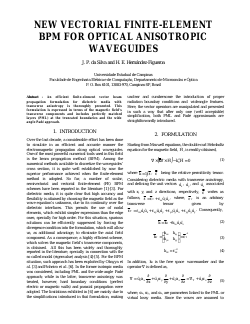##### New Vectorial Finite-Element BPM for Optical Anisotropic Waveguides

###### Abstract
"An efficient finite-element vector beam propagation formulation for dielectric media with transverse anisotropy is thoroughly presented. This formulation is expressed in terms of the magnetic field\u2019s transverse components and includes perfectly matched layers (PML) at the truncated boundaries and the wide angle Pad\u00e9 approach."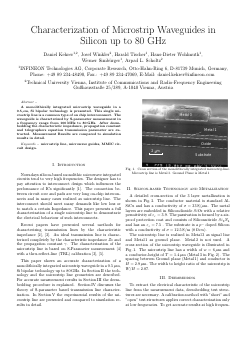##### Characterization of Microstrip Waveguides in Silicon up to 80 GHz

###### Abstract
"A monolithically integrated microstrip waveguide in a 0.5 \u00b5m, Si bipolar technology is presented. This single microstrip line is a common type of on chip interconnnect. The waveguide is characterized by S-parameter measurement in a frequency range from 100 MHz to 80 GHz. After deembedding the characteristic impedance, propagation constant and telegraphers equation transmission parameter are extracted. Measurement Results are compared to simulation results in detail."

Página de 19